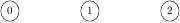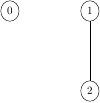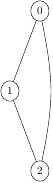Queries for Graphs: search statistic / browse statistics / browse maps from / browse maps to

# 1. Definition & Example

• A (finite, undirected, simple) graph $G = (V,E)$ consists of a finite set $V$ of vertices and a set $E \subseteq \binom{V}{2}$ of edges.

• Two graphs $G = (V,E)$ and $G = (V',E')$ are isomorphic if there is a bijection $\psi : V \rightarrow V'$ such that

• $$\{u,v\} \in E \Leftrightarrow \{\psi(u),\psi(v)\} \in E'.$$

• Unlabelled graphs on $n$ vertices are graphs up to graph-isomorphism.

• A canonical form of an unlabelled graph is a relabelling of the vertices (an isomorphic graph) such that any two graphs are isomorphic if and only if they have the same canonical form.

 the 4 Graphs of size 3([],3) ([(1,2)],3) ([(0,2),(1,2)],3) ([(0,1),(0,2),(1,2)],3)
• Unlabelled graphs are graphically represented by their unlabelled vertices with edges connecting adjacent vertices.
• For the number of unlabelled graphs see OEIS:A000088.

# 2. Further definitions

• If $\{u,v\}$ is an edge in a graph $G$, then $u$ and $v$ are adjacent vertices. $u$ and $v$ are also known as neighbors. The set of neighbors of $v$, denoted $N(v)$, is called the neighborhood of $v$. The closed neighborhood of $v$ is $N[v]=N(v)\cup {v}$.

• If two edges share a vertex in common (e.g. $\{u,v\}$ and $\{v,w\})$, then they are adjacent edges.

• The degree of a vertex $v$, denoted deg($v$), is the number of vertices adjacent to $v$.

• We call $|V(G)|$, the cardinality of the vertices of a graph $G$, the order of the graph. We also say $|E(G)|$, the cardinality of the edges of a graph $G$, is the size of the graph.

• A graph of size 0 is called an empty graph. Any graph with at least one edge is called nonempty.

• A graph is complete when any two distinct vertices are adjacent. The complete graph of $n$ vertices is notated $K_{n}$

• A planar graph is a graph that can be embedded in the plane. I.e. it can be drawn on the plane such a way that its edges intersect only at their endpoints.

• A walk $W$ in a graph $G$ is a sequence of vertices in $G$, beginning at a vertex $u$ and ending at a vertex $v$ such that the consecutive vertices in $W$ are adjacent in $G$.

• A walk whose initial and terminal vertices are distinct is called an open walk, otherwise it is a closed walk.

• A walk in which no edge repeats is called a trail.

• A path $P$ in a graph $G$ is a sequence of edges which connect a sequence of vertices which, are all distinct from one another. A path can also be thought of as a walk with no repeated vertex.

• A simple path is one which contains no repeated vertices (in other words, it does not cross over itself).

• If there is a path from a vertex $u$ to a vertex $v$ then these two vertices are said to be connected. If every two vertices in a graph $G$ are connected, then $G$ is itself a connected graph.

• A nontrivial closed walk in a graph $G$ in which no edge is repeated is a circuit in $G$.

• A circuit with vertices $v_1, v_2, ..., v_k, v_1$ where $v_2, ..., v_k$ are all distinct is called a cycle.

• Let $G$ be a nontrivial connected graph. A circuit $C$ of $G$ that contains every edge of $G$ (necessarily exactly once) is called an Eulerian Circuit. Any graph which contains an Eularian Circuit is called Eularian. A graph is Eulerian if and only if all its vertices have even degrees.

A nontrivial connected graph $G$ is Eularian if and only if every vertex of G has even degree.

• Every planar graph is four-colorable. That is, the chromatic number of a planar graph is at most four.

• G. Chartrand, L. Lesniak, and P. Zhang. Graphs and Digraphs. CRC Press, Oct. 2010.

# 5. Technical information for database usage

• A graph is uniquely represented as a tuple (E,n) where E is the sorted list of edges in the canonical labelling and n is the number of vertices.

• Graphs are graded by the number of vertices.
• The database contains all graphs of size at most 7.